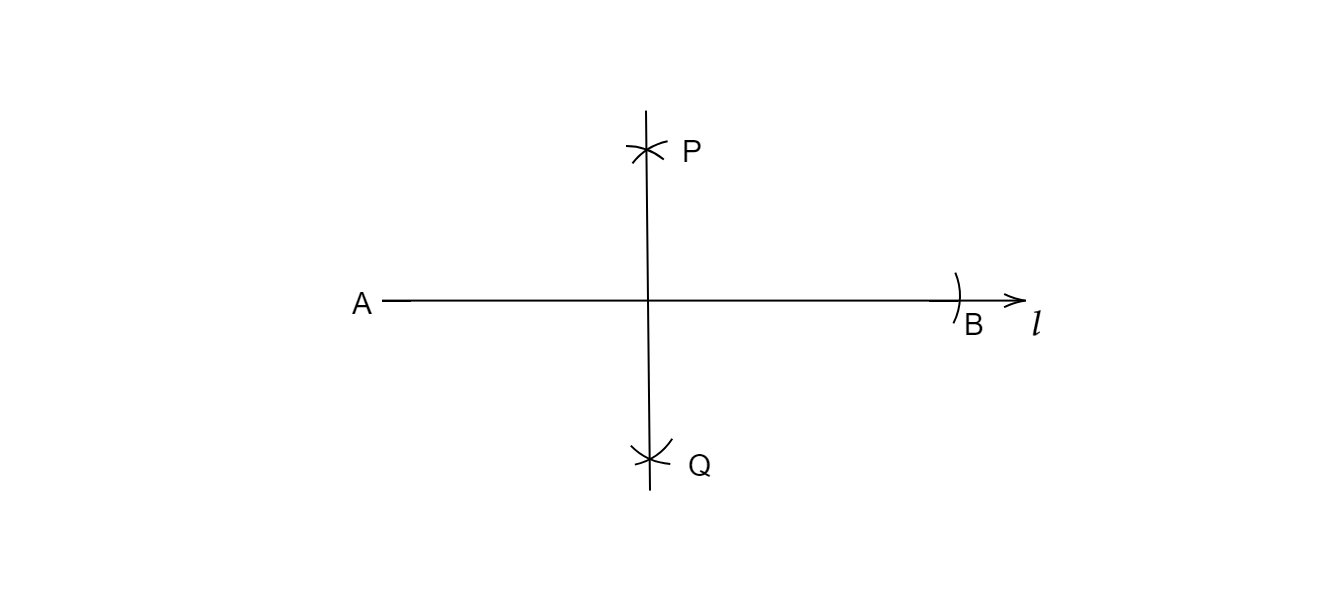# Draw $\overline{A B}$ of length $7.3 \mathrm{~cm}$ and find its axis of symmetry.

Given:

$\overline {AB}=7.3\ cm$.

To do:

We have to draw $AB$ of length $7.3\ cm$ and find its axis of symmetry.

Solution:

Let's first draw a line segment of length $7.3\ cm$.

1. Draw a line $l$, mark point $A$ on it.

2. Since length is $7.3\ cm$, we measure $7.3\ cm$ using ruler and compass.3. Now keeping compass opened the same length, we keep pointed end at point A and draw an arc on line l.

So, the required line segment is $AB =7.3\ cm$.

Now, we need to find its axis of symmetry.

1. Given a line segment $AB$.

2. Now with $A$ as centre and radius more than half $AB$, draw an arc on top and bottom of $AB$.

3. Now with $B$ as the centre and same radius, draw an arc on top and bottom of $AB$.

4. Where the two arcs intersect above $AB$ is point $P$ and where the two arcs intersect below $AB$ is point $Q$.

Join $PQ$.

$PQ$ is the line of symmetry of line segment AB.

Updated on: 10-Oct-2022

27 Views